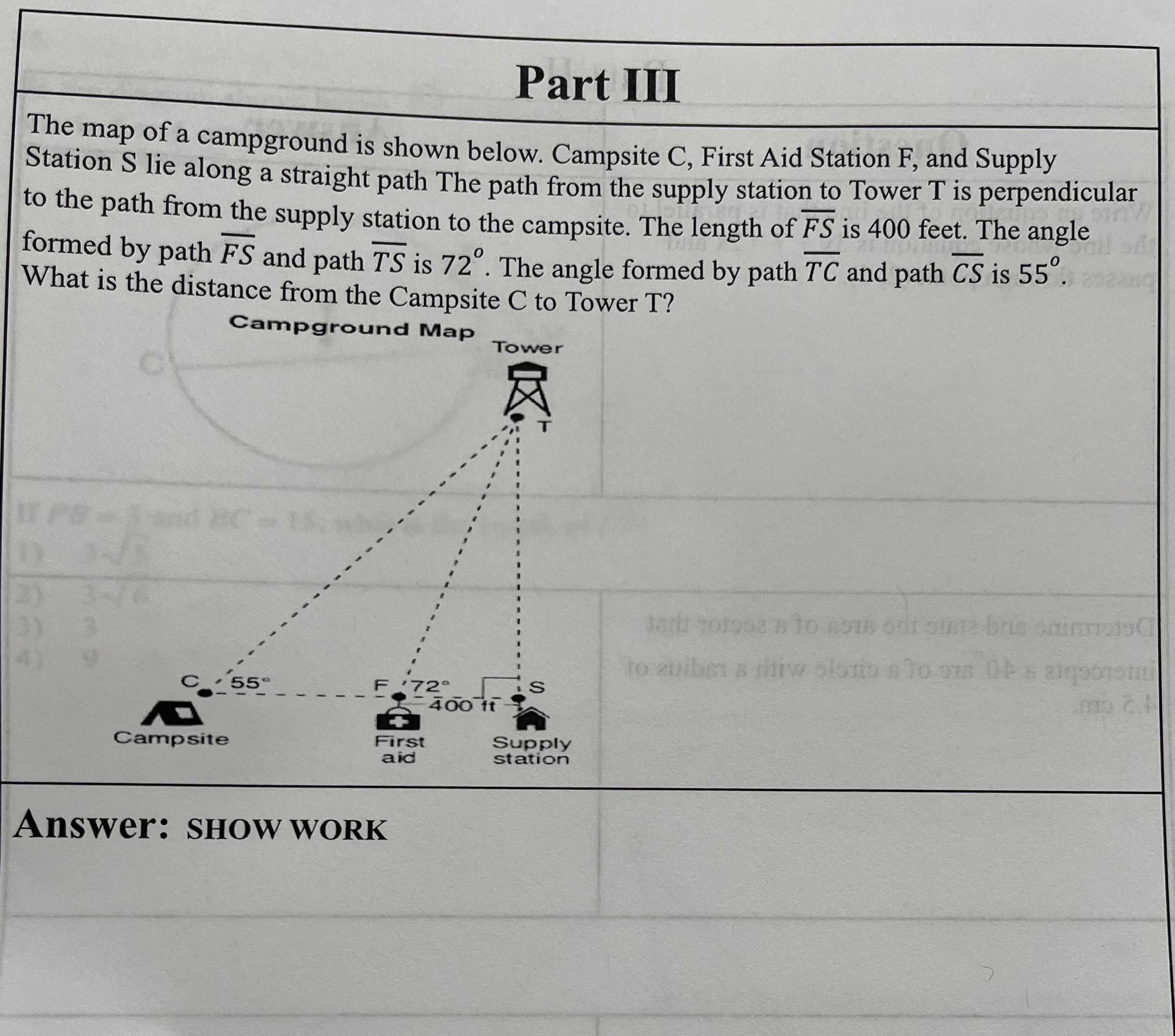### Still have math questions?The map of a campground is shown below. Campsite C, First Aid Station F, and Supply Station S lie along a straight path The path from the supply station to Tower T is perpendicular to the path from the supply station to the campsite. The length of $$\overline { F S }$$ is $$400$$ feet. The angle formed by path $$\overline { F S }$$ and path $$\overline { T S }$$ is $$72 ^ { \circ }$$ . The angle formed by path $$\overline { T C }$$ and path $$\overline { C S }$$ is $$55 ^ { \circ }$$ . What is the distance from the Campsite C to Tower T?